## copula package

# load packages
libs<-c("copula","scatterplot3d")
invisible(lapply(libs, library, character.only = TRUE))

Elliptical and Archimedean copulas can be defined using the copula class.

### Elliptical Copulas

First, we create a normalCopula object with 2 dimensions and (exchangeable) correlation of $$\rho=0.4$$

set.seed(1)

# create normalCopula object with 2 dimensions and exchangeable correlation
myCop.norm <- ellipCopula(family="normal", dim=2, dispstr="ex", param = 0.4)

# generate random draws from copula
u <- rCopula(1000, myCop.norm)

After generating $$n=1000$$ random draws from the Normal copula, we check the correlation and produce a scatter plot of the draws

# check correlation
cor(u)
##           [,1]      [,2]
## [1,] 1.0000000 0.3995705
## [2,] 0.3995705 1.0000000
# scatter plot
plot(u[,1],u[,2], main="1000 draws from Normal copula")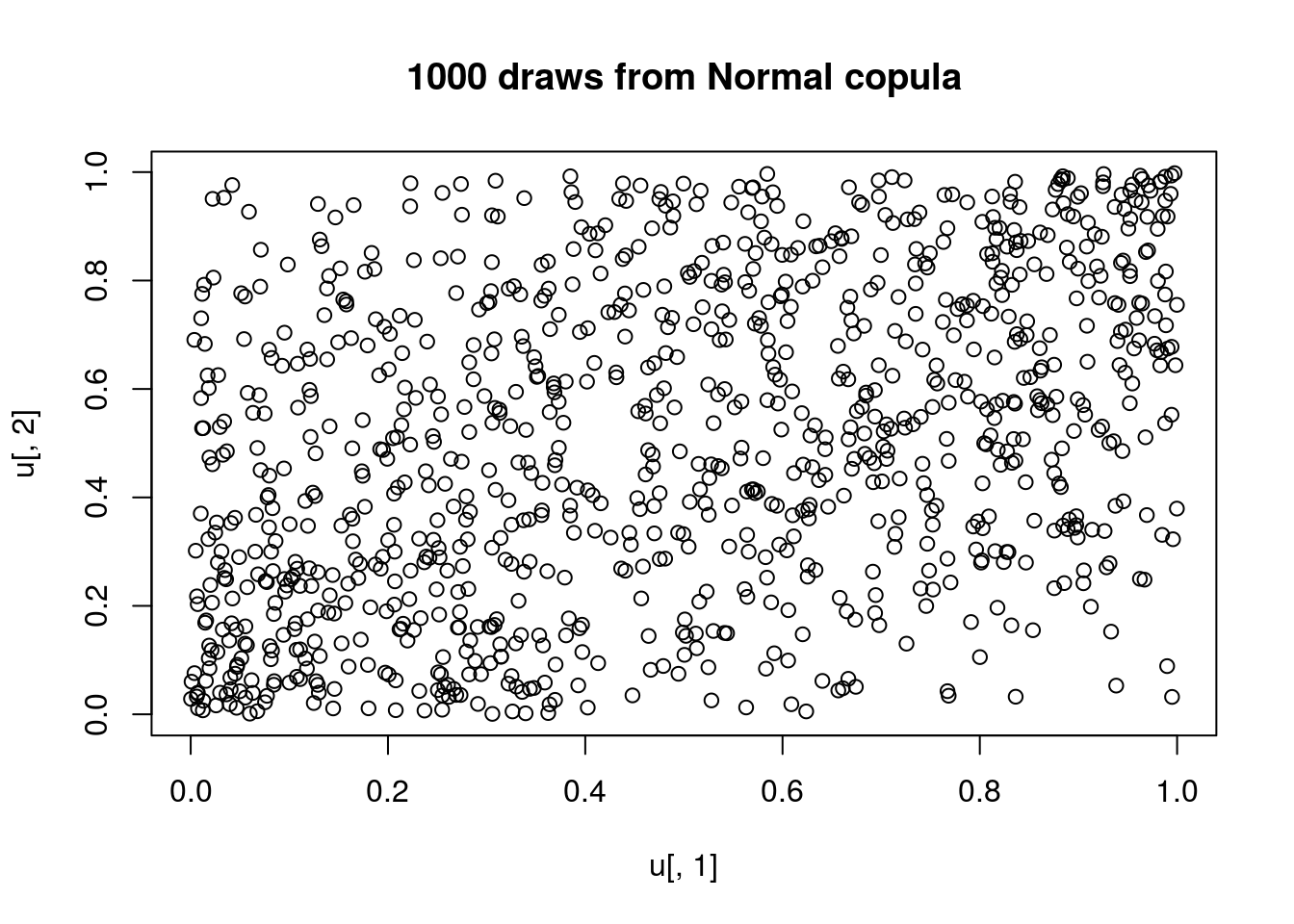We can also produce contour and perspective plots of the copula density and distribution functions

# contour and perspective plot of density function
contour(myCop.norm,dCopula, main="contour plot of Normal copula density")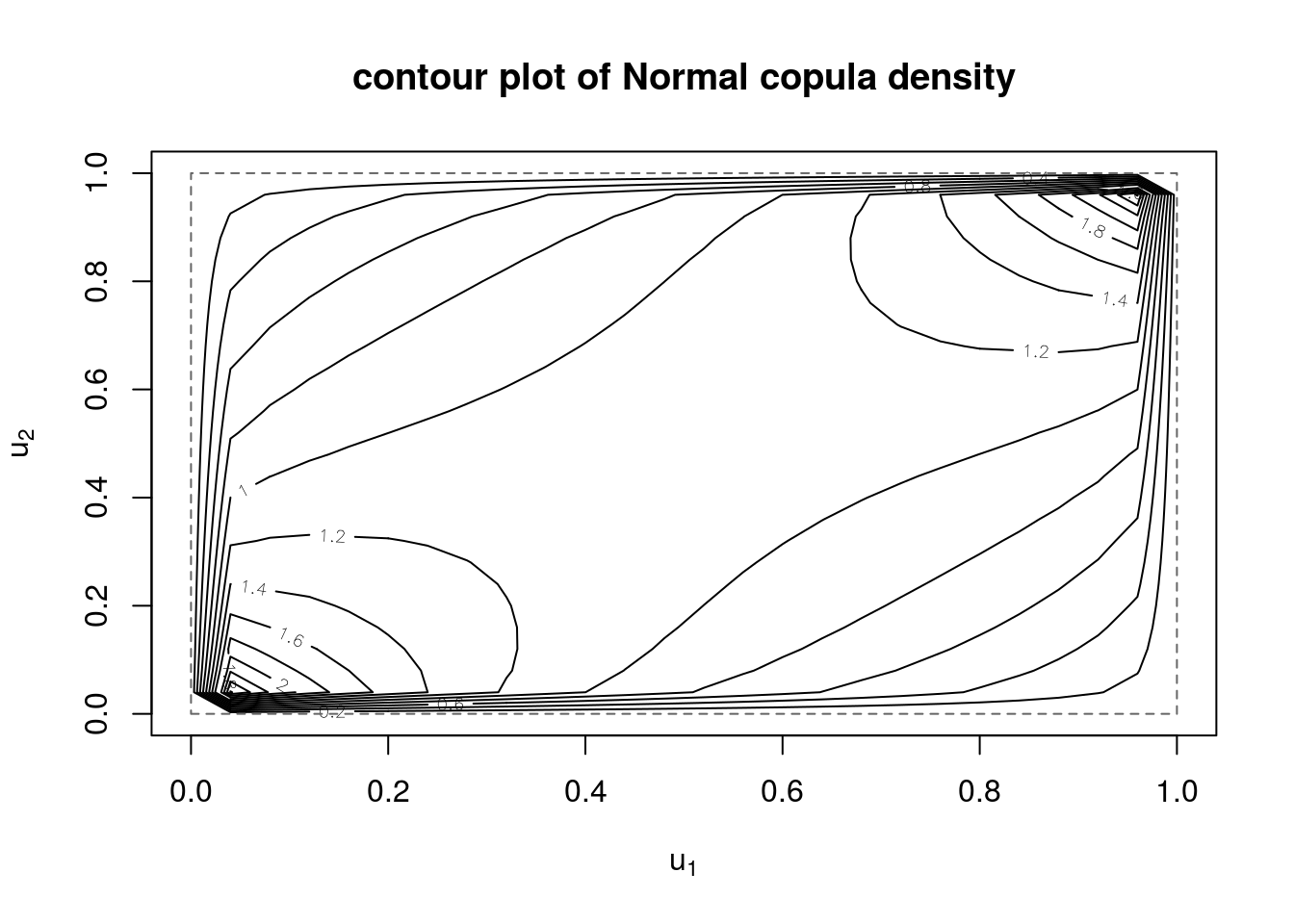persp(myCop.norm,dCopula, main="perspective plot of Normal copula density")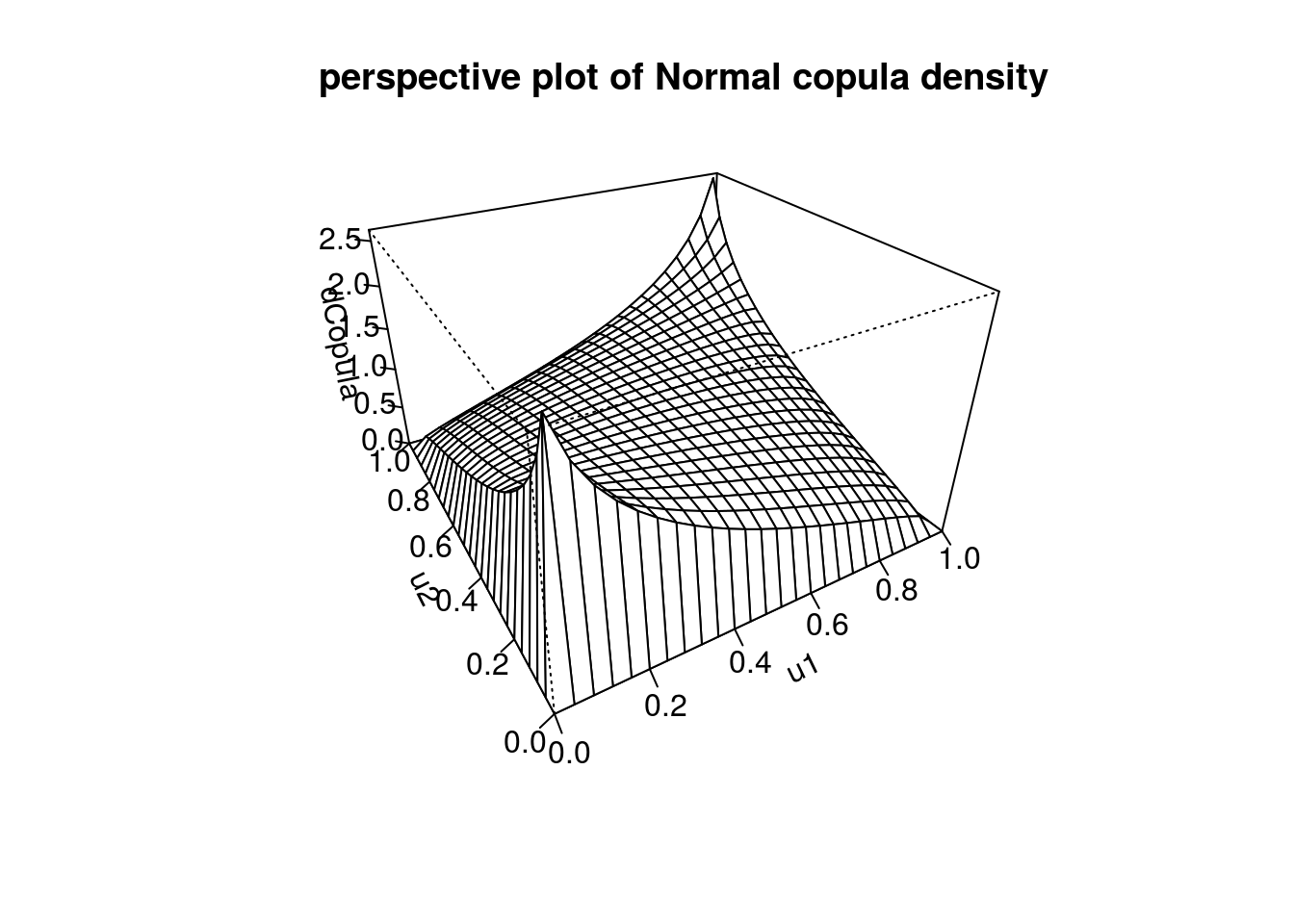# contour and perspective plot of distribution function
contour(myCop.norm,pCopula, main="contour plot of Normal copula distribution")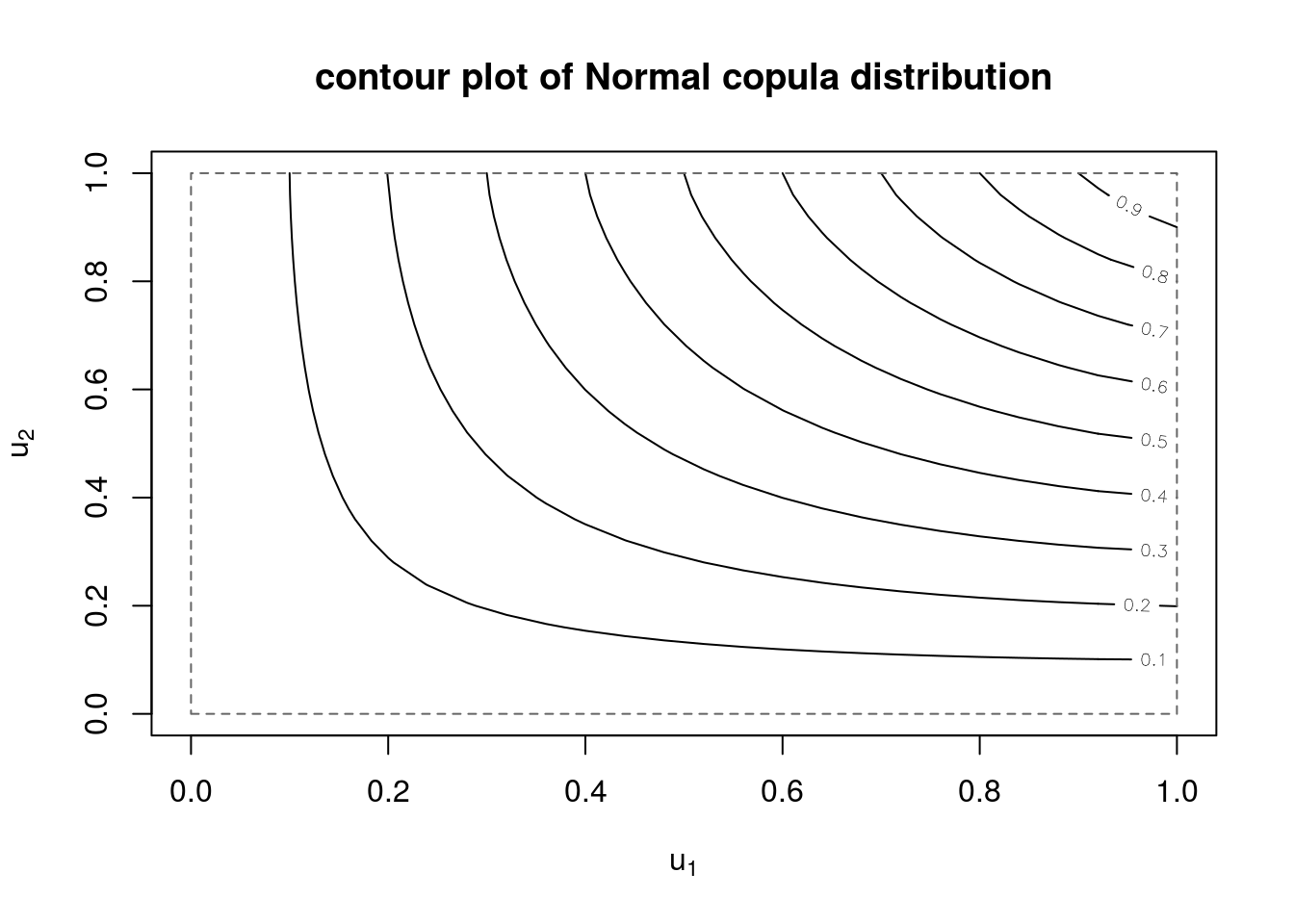persp(myCop.norm,pCopula, main="perspective plot of Normal copula distribution")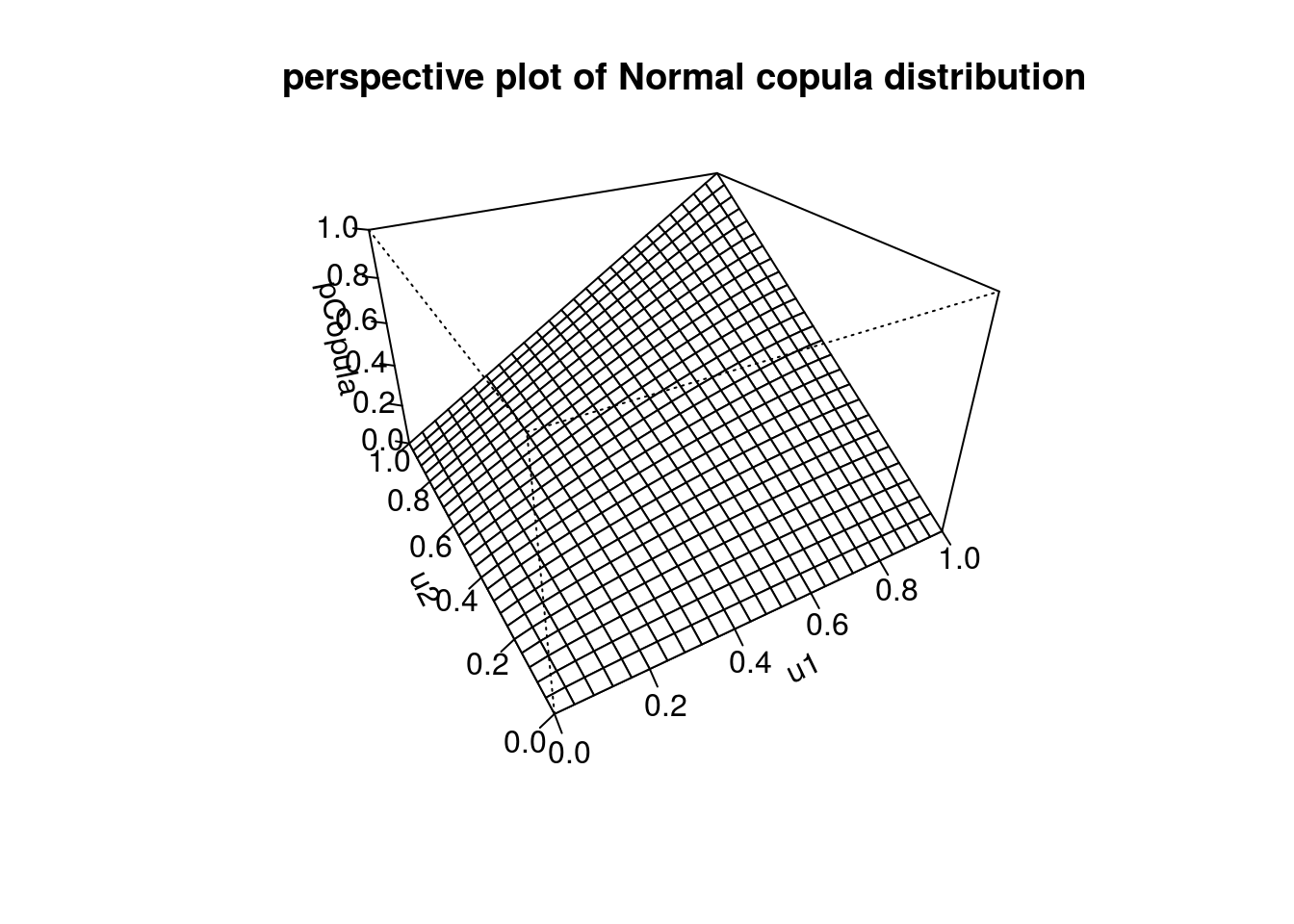Another common elliptical copula is the $$t$$. We create a tCopula model with 8 degrees of freedom and 3 dimensions and specify a banded (Toeplitz) correlation structure with parameters $$\rho_1=0.8$$ and $$\rho_2=0.5$$.

# create tCopula object with 3 dimensions and banded (toeplitz) correlation and 8 df
myCop.t <- ellipCopula(family="t", dim=3, dispstr="toep", param = c(0.8, 0.5), df=8)

We draw $$n=1000$$ samples from this copula and verify that the marginals are uniform and the correlation is correct

v<-rCopula(1000, myCop.t)

par(mfrow=c(1,3), mar=c(2,2,1,1))
hist(v[,1])
hist(v[,2])
hist(v[,3])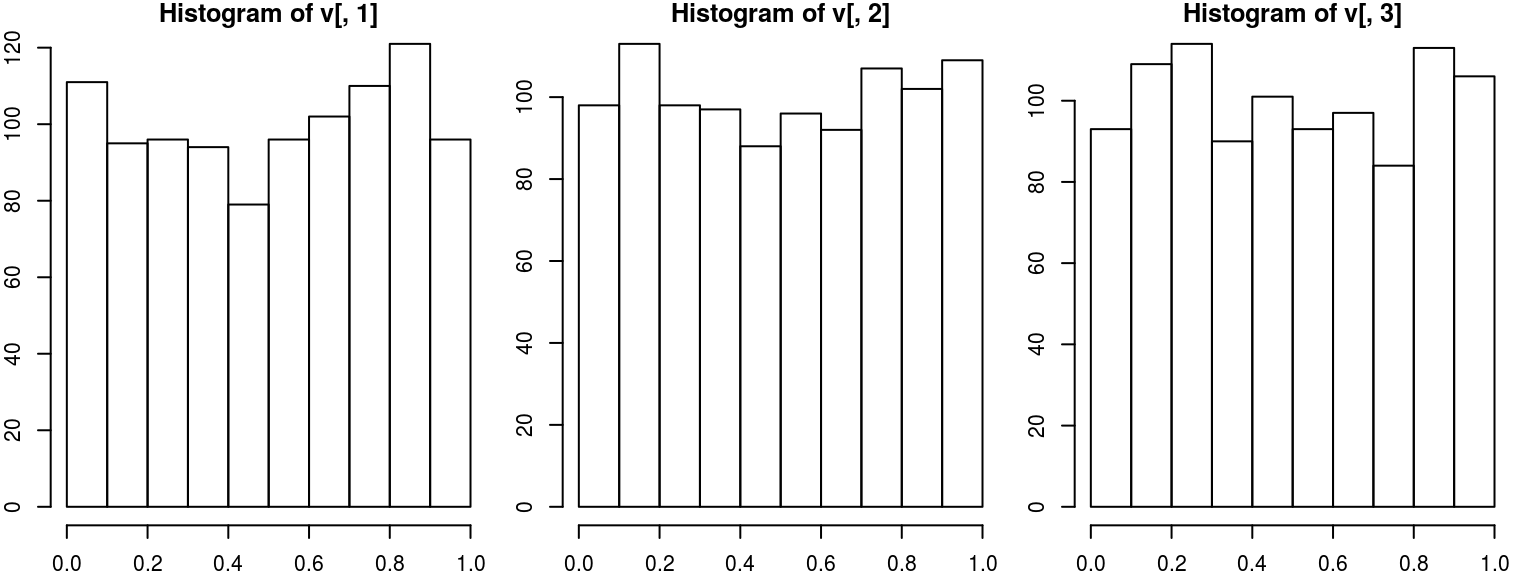#check correlation
cor(v)
##           [,1]      [,2]      [,3]
## [1,] 1.0000000 0.7963426 0.4895844
## [2,] 0.7963426 1.0000000 0.7870277
## [3,] 0.4895844 0.7870277 1.0000000

We can also produce a 3-d scatterplot of a random subset the samples (to avoid overcrowding the plot) and evaluate the copula density and distribution at the sampled values.

# scatter plot
par(mfrow=c(1,1))
subsamp <- sample(1:nrow(v),100)
scatterplot3d(v[subsamp,], type="h")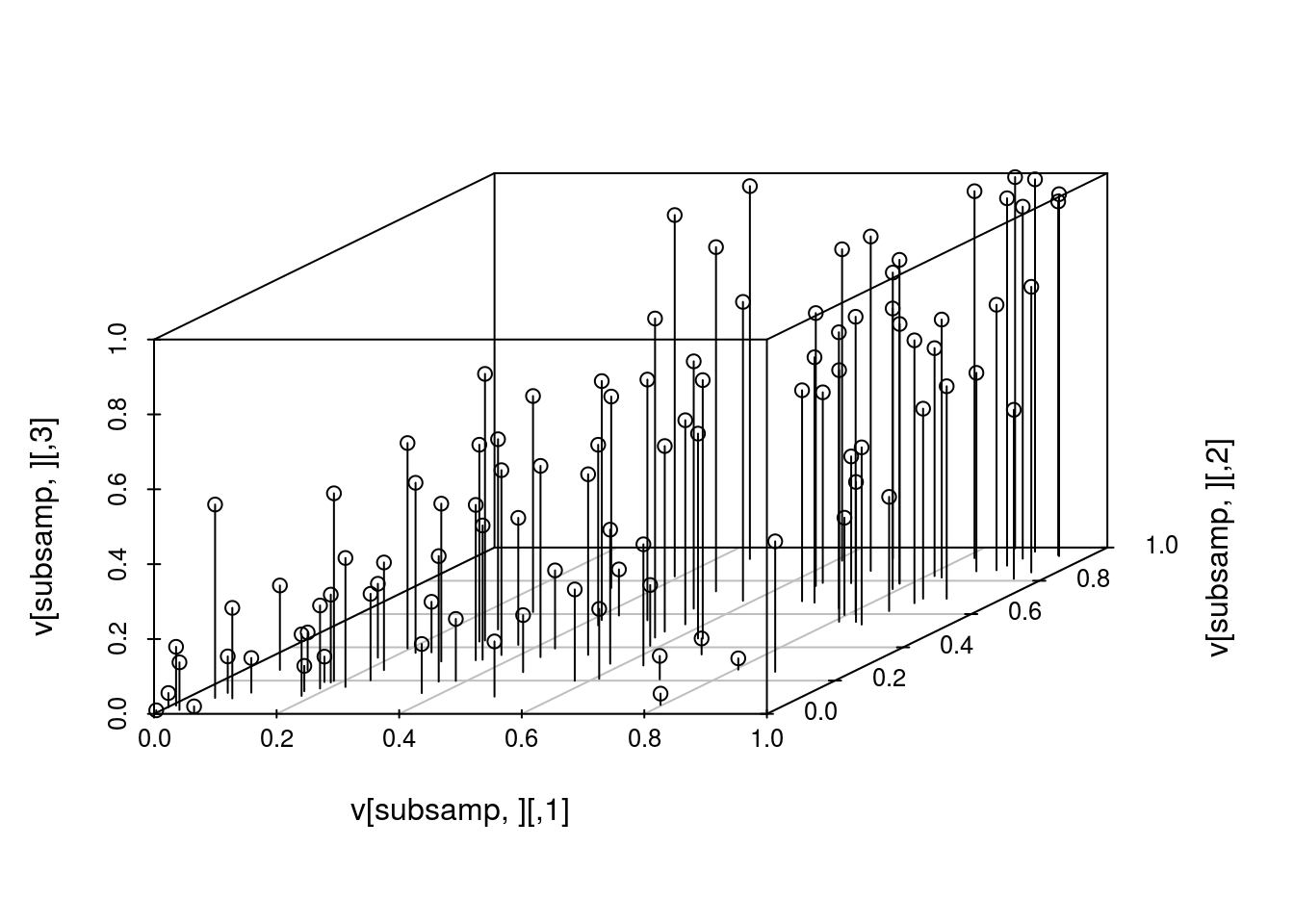#evaluate density
head(dCopula(v,myCop.t), 10)
##   0.2684407 4.3166171 9.8703657 4.4894340 2.0922633 1.5403350 0.6653583
##   3.5466681 0.2271933 3.1185452
#evaluate distribution P(U1 <= u1, U2 <= u2, U3 <= u3)
head(pCopula(v,myCop.t), 10)
##   0.01283143 0.67535588 0.02485953 0.11549992 0.58492358 0.19596911
##   0.19517213 0.10670446 0.37418632 0.03529708

### Archimedean Copulas

Archimedean copulas are another popular choice. We create 3 dimensional Clayton copula with $$\alpha=2$$ and a 2 dimensional Frank copula with $$\alpha=5$$.

myCop.clayton <- archmCopula(family = "clayton", dim = 3, param = 2)

myCop.frank <- archmCopula(family = "frank", dim = 2, param = 5)

Contour plots for the Frank copula density and distribution are shown below.

par(mfrow=c(1,2))
contour(myCop.frank, dCopula, main="contour plot of Frank copula density", box01=FALSE)
contour(myCop.frank, pCopula, main="contour plot of Frank copula distribution", box01=FALSE)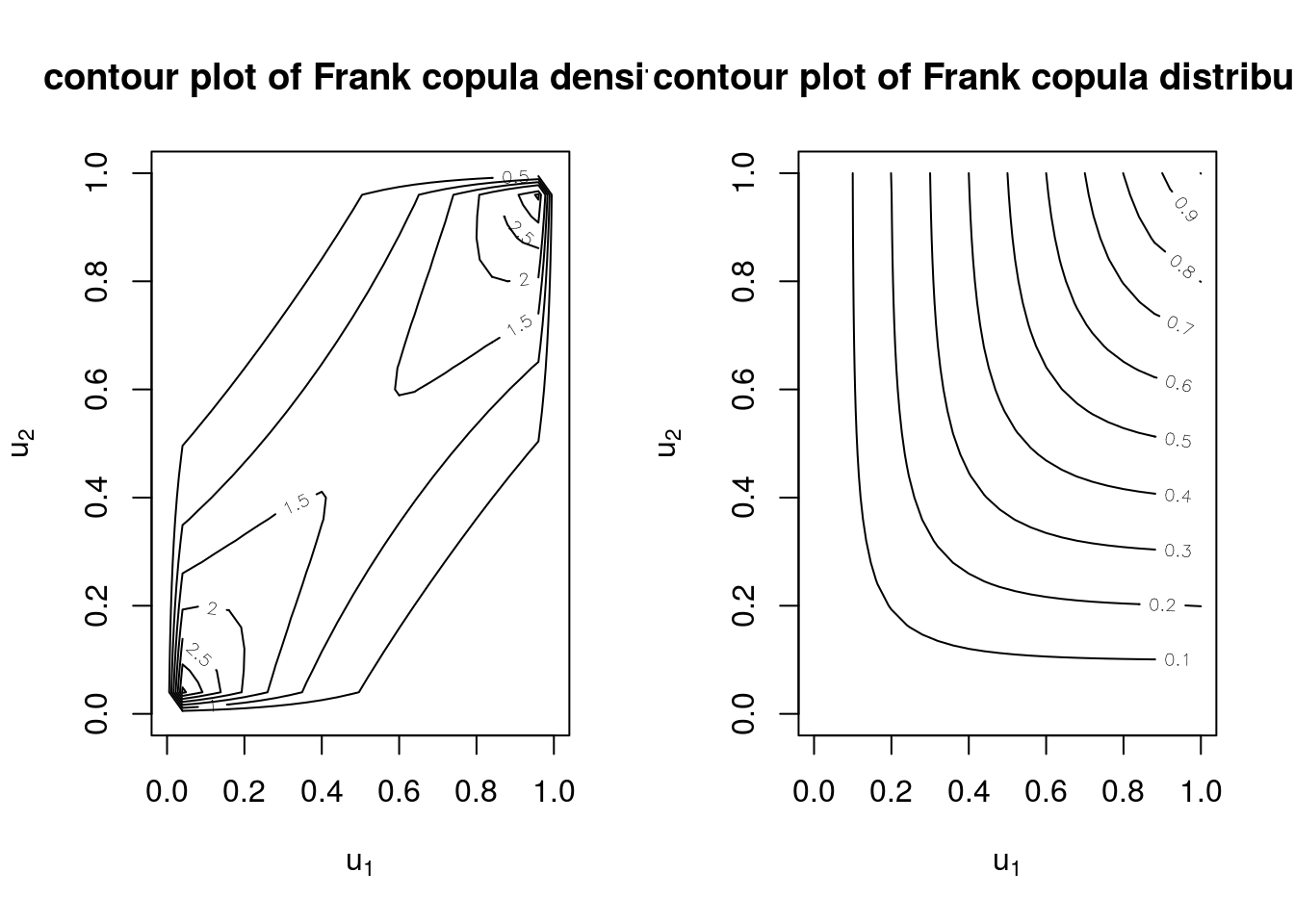### Building multivariate distributions using copulas

Given a copula and marginal distribution functions, the mvdc class defines a multivariate distribution function.

We create a multivariate distribution function by combining 3 normal marginal distributions ($$N(0,2^2)$$, $$N(0,1^2)$$, and $$N(1,3^2)$$) with a 3-dimensional gumbel copula with $$\alpha=3$$. We then draw 1000 samples from this mulivariate distribution and check the marginal means and sds.

myMvd <- mvdc(copula = archmCopula(family="gumbel", dim=3, param=3),
margins = c("norm", "norm", "norm"),
paramMargins = list(list(mean=0, sd = 2),
list(mean=0, sd = 1),
list(mean=1, sd = 3)))

# generate random draws from multivariate distribution
w<-rMvdc(1000, myMvd)

# check marginal mean and sd
apply(w,2, function(x) c(mean(x),sd(x)) )
##              [,1]        [,2]      [,3]
## [1,] -0.004235106 0.001274415 0.9862673
## [2,]  1.931454581 0.971024048 2.9104349

In addition, we can evaluate the density and distribution at the sampled points

# evaluate density
head(dMvdc(w,myMvd), 10)
##   0.060681635 0.050706591 0.039972322 0.015332861 0.002910626
##   0.011808811 0.031937764 0.022124490 0.007403489 0.012785177
# evaluate distribution
head(pMvdc(w,myMvd), 10)
##   0.71825269 0.48390858 0.18002531 0.19355349 0.66116464 0.98823123
##   0.69735867 0.56023835 0.33979564 0.09292659

Next, we make a 2-dimensional distribution from normal marginals ($$N(0,3)$$ and $$N(0,4)$$) and Clayton copula with $$\alpha = 2$$ and show the contour and perspective plots of the density and distribution.

myMvd1 <- mvdc(copula = archmCopula(family = "clayton", param = 2),
margins = c("norm", "norm"),
paramMargins = list(list(mean=0, sd = 3),
list(mean=0, sd = 4)))

# contour and perspective plots of density
contour(myMvd1, dMvdc, xlim=c(-10,10), ylim=c(-10,10), nlevels=10,
main="contour plot of 2-d density function\n (Clayton copula, Normal marginals)")persp(myMvd1, dMvdc, xlim=c(-10,10), ylim=c(-10,10),
main="perspective plot of 2-d density function\n (Clayton copula, Normal marginals)")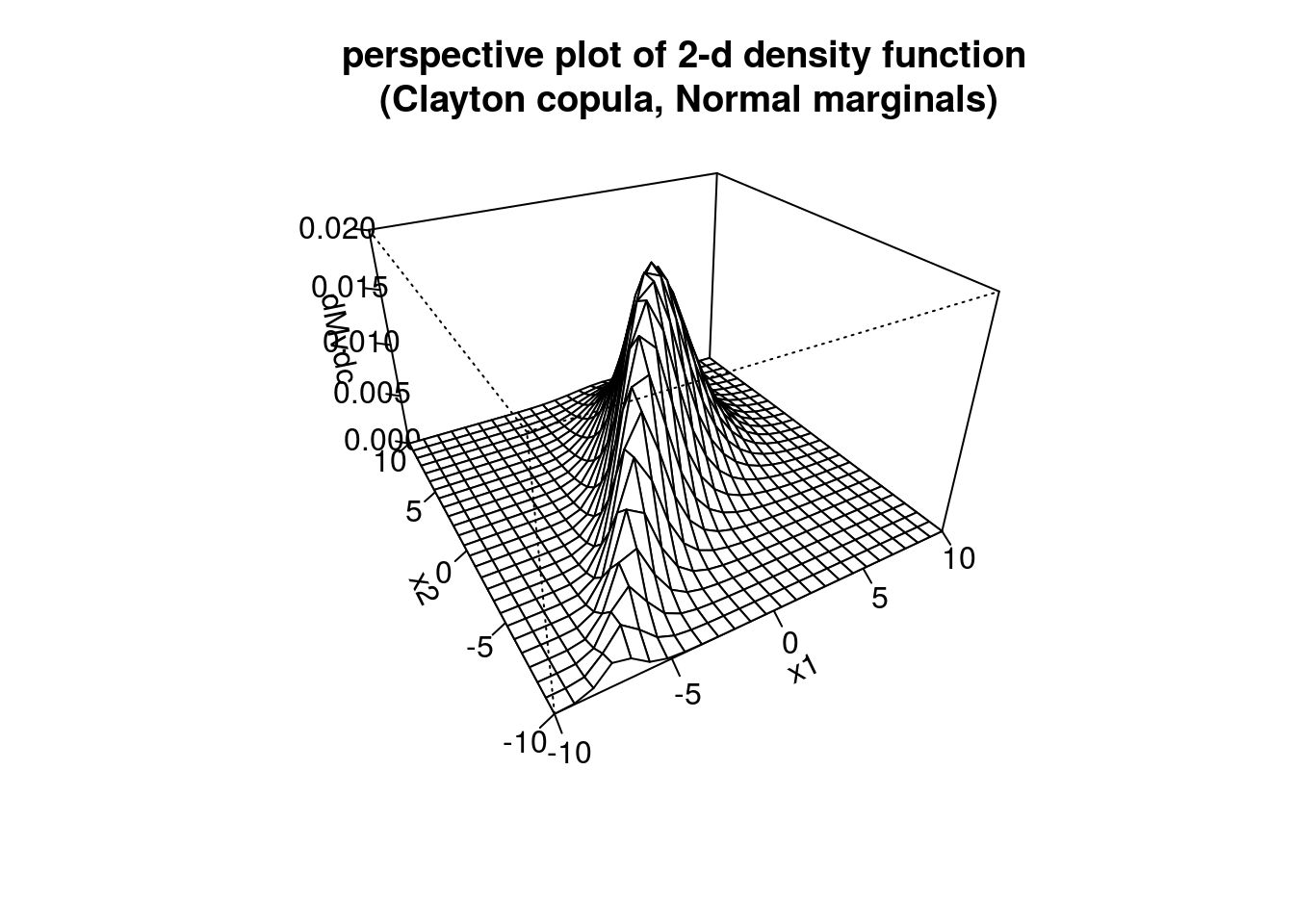# contour and perspective plots of distribution
contour(myMvd1, pMvdc, xlim=c(-10,10), ylim=c(-10,10), nlevels=10,
main="contour plot of 2-d distribution function\n (Clayton copula, Normal marginals)")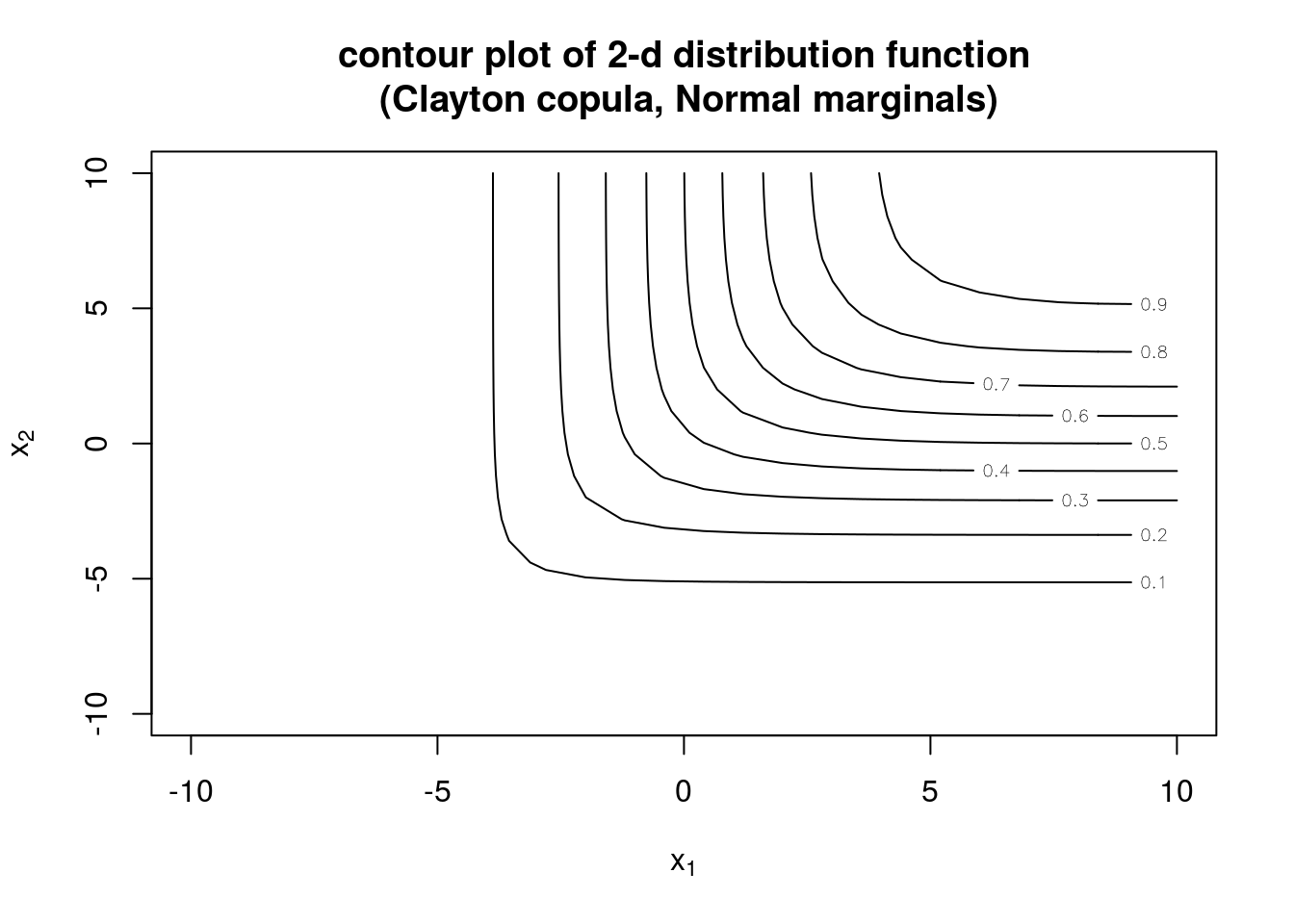persp(myMvd1, pMvdc, xlim=c(-10,10), ylim=c(-10,10),
main="perspective plot of 2-d distribution function\n (Clayton copula, Normal marginals)")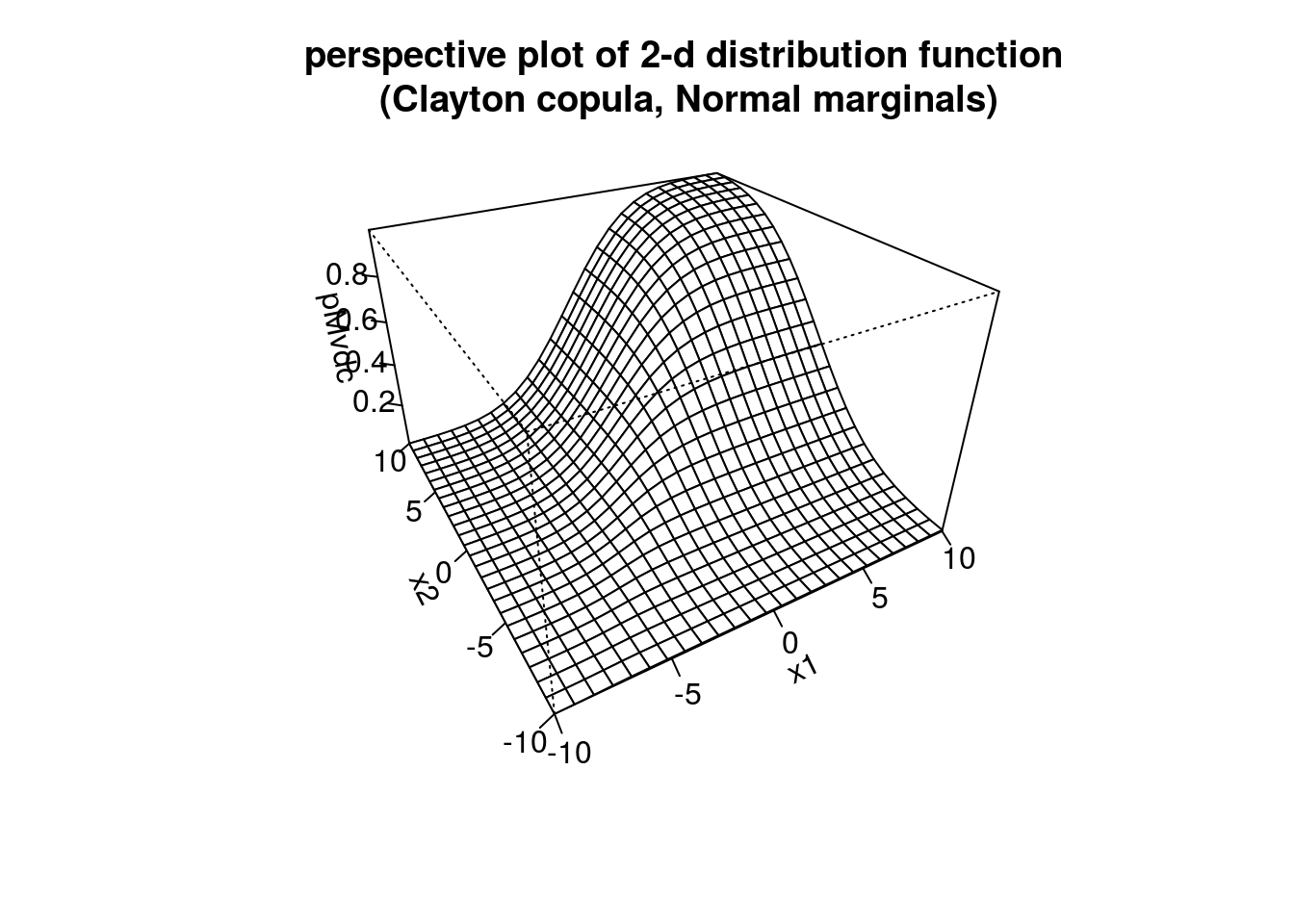### Fitting copula models

The copula package provides the functions loglikCopula() and loglikMvdc() to compute the log-likelihood of the copula and the multivariate copula-based distribution function. fitCopula() and fitMvdc() perform maximum likelihood estimation.

# simulate data
Mvd <- mvdc(copula = ellipCopula(family="normal", param=0.5),
margins = c("gamma","gamma"),
paramMargins = list(list(shape=2, scale=1),
list(shape=3, scale=2)))
dat <- rMvdc(200, Mvd)

# 'empty' mvdc object, i.e. parameters not specified
Mvd0 <- mvdc(copula = ellipCopula(family="normal"),
margins = c("gamma","gamma"),
paramMargins = list(list(shape=NA, scale=NA),
list(shape=NA, scale=NA)))

Mvd1 <- mvdc(copula = ellipCopula(family="normal", param=0.8),
margins = c("gamma","norm"),
paramMargins = list(list(shape=4, scale=3),
list(mean=0, sd=1)))

# loglikelihood w/ correct parameter values
loglikMvdc(c(2,1,3,2,0.5),dat,Mvd)
##  -788.8245

We calculate the MLEs …

Only the distributional assumptions (form of copula and marginals) of the mvdc class object are used when passed to fitMvdc(); the parameter values from the mvdc object are not used

# method of moments estimates to start optimization
mm <- apply(dat,2,mean)
vv <- apply(dat,2,var)
b1.0 <- c(mm^2/vv,vv/mm)
b2.0 <- c(mm^2/vv,vv/mm)
a.0 <- sin(cor(dat[,1], dat[,2], method="kendall")*pi/2)
start <- c(b1.0, b2.0, a.0)

# get MLE
fit <- fitMvdc(dat, Mvd, start = start,
optim.control = list(trace=TRUE, maxit = 2000))
## initial  value 787.745363
## final  value 787.113186
## converged
## initial  value 787.113186
## final  value 787.113186
## stopped after 1 iterations
fit
## Call: fitMvdc(data = dat, mvdc = Mvd, start = start, optim.control = list(trace = TRUE,
##     maxit = 2000))
## Maximum Likelihood estimation based on 200 2-dimensional observations.
## Copula:  normalCopula
## Margin 1 :
## m1.shape m1.scale
##    2.053    1.011
## Margin 2 :
## m2.shape m2.scale
##    3.427    1.713
## Copula:
##  rho.1
## 0.4947
## The maximized loglikelihood is -787.1
## Optimization converged
# w/ 'empty' mvdc object
fit0 <- fitMvdc(dat, Mvd0, start = start,
optim.control = list(trace=TRUE, maxit = 2000))
## initial  value 787.745363
## final  value 787.113186
## converged
## initial  value 787.113186
## final  value 787.113186
## stopped after 1 iterations
fit0
## Call: fitMvdc(data = dat, mvdc = Mvd0, start = start, optim.control = list(trace = TRUE,
##     maxit = 2000))
## Maximum Likelihood estimation based on 200 2-dimensional observations.
## Copula:  normalCopula
## Margin 1 :
## m1.shape m1.scale
##    2.053    1.011
## Margin 2 :
## m2.shape m2.scale
##    3.427    1.713
## Copula:
##  rho.1
## 0.4947
## The maximized loglikelihood is -787.1
## Optimization converged
# misspecified
fit1 <- fitMvdc(dat, Mvd1, start = start,
optim.control = list(trace=TRUE, maxit = 2000))
## initial  value 1201.877971
## iter  10 value 810.919579
## iter  20 value 800.683656
## iter  20 value 800.683651
## iter  20 value 800.683651
## final  value 800.683651
## converged
## initial  value 800.683651
## final  value 800.683651
## stopped after 1 iterations
fit1
## Call: fitMvdc(data = dat, mvdc = Mvd1, start = start, optim.control = list(trace = TRUE,
##     maxit = 2000))
## Maximum Likelihood estimation based on 200 2-dimensional observations.
## Copula:  normalCopula
## Margin 1 :
## m1.shape m1.scale
##    2.053    1.011
## Margin 2 :
## m2.mean   m2.sd
##   5.870   3.034
## Copula:
##  rho.1
## 0.4829
## The maximized loglikelihood is -800.7
## Optimization converged

For large $$p$$, we can use an alternative two-stage strategy called inference functions for margins (IFM). In step one IFM estimates the marginal parameters, $$\beta$$. In step two, estimates of the copula association parameters $$\alpha$$ given $$\hat{\beta}$$ are produced. Also called two-stage parametric ML method in censored data setting Note that the SE of $$\hat{\alpha}$$ is an underestimate since the variability in $$\hat{\beta}$$ is not corectly accounted for.

We can obtain a consistent estimate of $$\alpha$$ using canonical ML (CML) which uses the empirical CDFs of each marginal to transform the observations into pseudo-observations. The pseudo-observations are used to estimate $$\hat{\alpha}_{CML}$$

TO incorporate covariates into the margins, we need the log-likelihood function which is the summation of the copula log-likelihood and the log-likelihood of all marginals

(ref equation 9)

# example from appendix A